# Arithmetic function

## Definition

An arithmetic function is a function from the set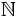of natural numbers to a commutative unital ring, i.e., a commutative ring with.

Typically, arithmetic functions are to the ring of integers, though they are sometimes to bigger rings such as the field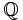of rational numbers or to the field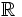of real numbers.

## Structure

### Dirichlet product

Further information: Dirichlet product

The Dirichlet product of two arithmetic functionsis defined as:.

The Dirichlet product is a commutative and associative operation, and any function such that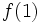is a unit is a unit with respect to the Dirichlet product function. The identity for the Dirichlet product is the functionthat is the indicator function of: it sendsto, and everything else to.

The multiplicative functions form a subgroup of the group of invertible Dirichlet functions.

### Pointwise product

Given two arithmetic functions, we can take the pointwise product of these functions. This is defined as: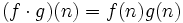.

Pointwise product is commutative and associative on arithmetic functions. The identity for pointwise product is the all ones function, i.e., the function that sends every natural number to. A function is invertible with respect to the pointwise product if and only if the value it takes at every natural number is invertible.

### Composition

Composition of arithmetic functions does not in general make sense, because arithmetic functions take value, not in the natural numbers, but in an arbitrary commutative unital ring. However, in some cases, the commutative unital ring has characteristic zero, and hence contains a copy of the natural numbers. Further, some arithmetic functions take only positive integer values. In this case, we can compose two such arithmetic functions.

The identity element for composition is the identity function, typically denoted. This function sends every natural number to itself.

## Dirichlet series

Further information: Dirichlet series

For an arithmetic functionto a field of characteristic zero, the corresponding Dirichlet series is the formal sum:.

The Dirichlet series behaves particularly well with respect to Dirichlet products: the series for the Dirichlet product of two arithmetic functions is the same as the product of their Dirichlet series.

The Dirichlet series also has alternative, more compact, expressions for multiplicative functions and completely multiplicative functions.

## Properties

For a complete list of properties, refer:

### Completely multiplicative function

Further information: Completely multiplicative function

A completely multiplicative function can be thought of as an arithmetic function that is a unital homomorphism from the monoid of natural numbers to the multiplicative monoid of the commutative unital ring. More explicitly, it is a functionsuch that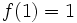and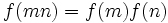for natural numbersand.

The pointwise product of completely multiplicative functions is completely multiplicative. A composition of completely multiplicative functions is also completely multiplicative, when it makes sense. However, a Dirichlet product of completely multiplicative functions need not be completely multiplicative.

### Multiplicative function

Further information: Multiplicative function

An arithmetic functionis termed multiplicative ifand, wheneverandare relatively prime,. Any completely multiplicative function is multiplicative, but the converse is not true.

The pointwise product of multiplicative functions is multiplicative, and the Dirichlet product of multiplicative functions is multiplicative. However, a composition of multiplicative functions, even when it makes sense, need not be multiplicative. This is becauseandbeing relatively prime does not implyandare relatively prime.

## Generalizations

### Generalization based on associate classes of elements

One direction of generalization is to think of the natural numbers as representatives of associate classes of nonzero elements in the ring of integers. In other words, every natural numberis the representative of the associate class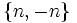in the ring of integers (where an associate class means an equivalence class under multiplication by the group of uniuts). Thus, an arithmetic function can also be thought of as a function on associate classes of elements in the ring of integers.

With this definition, we can generalize to any integral domain as follows: an arithmetic function on an integral domain is a function from associate classes of elements of the integral domain to a commutative unital ring. The generalization is typically done for unique factorization domains.

### Generalization based on ideals

Another direction of generalization is to think of the natural numbers as corresponding to nonzero ideals in the ring of integers. In other words, a natural numbercorresponds to the ideal.

With this definition, we can generalize to any integral domain as follows: an arithmetic function on an integral domain is a function from nonzero ideals of the integral domain to a commutative unital ring. The generalization is typically done for Dedekind domains, and more specifically, for the ring of integers in a number field.

Note that, for a principal ideal domain, associate classes of nonzero elements correspond to the nonzero ideals, so these two generalizations coincide.